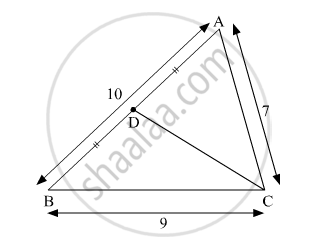Advertisement Remove all ads

# In ∆Abc, Ab = 10, Ac = 7, Bc = 9 Then Find the Length of the Median Drawn from Point C to Side Ab - Geometry

Sum

In ∆ABC, AB = 10, AC = 7, BC = 9 then find the length of the median drawn from point C to side AB

Advertisement Remove all ads

#### SolutionIn ∆ACB, point D is the midpoint of side AB.

$AD = BD = \frac{1}{2}AB = 5$

${CA}^2 + {CB}^2 = 2 {DC}^2 + 2 {AD}^2 \left( \text{by Apollonius theorem} \right)$

$\Rightarrow 7^2 + 9^2 = 2 {DC}^2 + 2\left( 5^2 \right)$

$\Rightarrow 49 + 81 = 2 {DC}^2 + 2\left( 25 \right)$

$\Rightarrow 130 = 2 {DC}^2 + 50$

$\Rightarrow 2 {DC}^2 = 130 - 50$

$\Rightarrow 2 {DC}^2 = 80$

$\Rightarrow {DC}^2 = 40$

$\Rightarrow DC = 2\sqrt{10}$

Hence, the length of the median drawn from point D to side AB is $2\sqrt{10}$

Is there an error in this question or solution?
Advertisement Remove all ads

#### APPEARS IN

Balbharati Mathematics 2 Geometry 10th Standard SSC Maharashtra State Board
Chapter 2 Pythagoras Theorem
Practice Set 2.2 | Q 2 | Page 43
Advertisement Remove all ads
Advertisement Remove all ads
Share
Notifications

View all notifications

Forgot password?